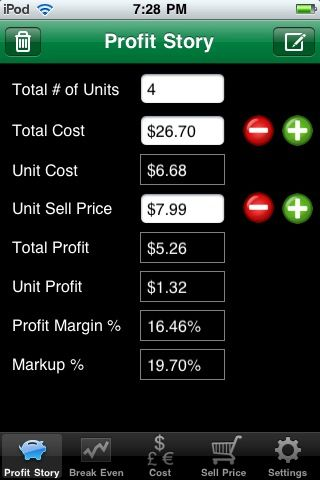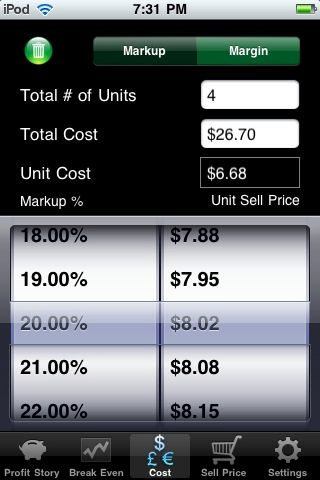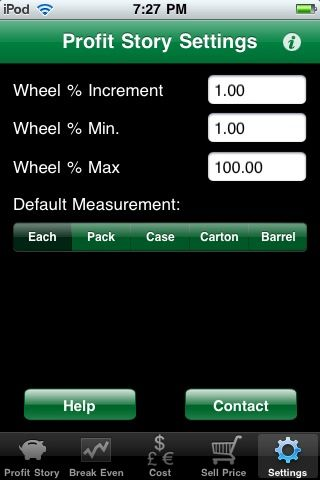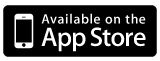Profit Story is a profit calculator that allows you to easily calculate Profit Margins, Markups, Suggested Sell Price and Suggested Cost Price information.  It also allows you to calculate your break even % and projected volume lift needed. Quickly see the impact of changing one measure on the other values.

Functions:
• Calculate Case and Unit Cost Price
• Calculate Unit Sell Price
• Calculate Unit Gross Profit Dollars
• Calculate Case Gross Profit Dollars
• Calculate Profit Margin %
• Calculate Markup %
• Calculate Break Even %
• Compare Current Cost, Sell Price, Gross Profit Dollars, Profit Margin % and Markup %, with proposed new pricing information in the Break Even analysis
• Easy view of Margin/Markup and Unit Sell Price using an interactive Price Wheel
• Set defaults for standard information
• Email and/or copy results for additional use of the information.

Profit Story